# Experimental, Analytical and ANSYS Computation of Novel Cascade Solar Desalination Still

Experimental, Analytical and ANSYS Computation of Novel Cascade Solar Desalination Still
Laboratoire de Mécanique Appliquée et Technologies - Centre de Recherche en Sciences et Technologies Industrielles et de la Santé, Mohammed V University in RABAT- ENSAM Rabat, Avenue de l’Armée Royale, BP 6207 Rabat, Morocco

Corresponding Author Email:
nidal_mouhsin@um5.ac.ma
Page:
397-404
|
DOI:
https://doi.org/10.18280/ijht.400206
29 June 2021
|
Accepted:
12 February 2022
|
Published:
30 April 2022
| Citation

OPEN ACCESS

Abstract:

This study presents a simple device using solar radiation as a source of energy to convert brackish water to freshwater. To enhance the productivity of the cascade of solar stills, a new structure of the absorber plate with sloped surfaces and baffles was proposed to present a slight water layer thickness and improve the amount of solar radiation incident. A physical model is developed taking into account various parameters which affect the distillate yield of solar sills. In the present work, the modeling of the cascade solar still uses ANSYS Fluent V18 to investigate the yield. A three-dimensional model was considered to simulate the temperature of the water, absorber plate, and freshwater productivity. A comparison was conducted based on the CFD simulation results, experimental and approximate analytical model data. It showed a reasonable agreement of the results obtained using the analytical model with the experimental and the simulation using ANSYS Fluent of different working parameters especially the productivity. The best performance was obtained on 15/07/2018, with a productivity rate of more than 1.67 kg/m2.hr.

Keywords:

desalination, brackish water, cascade solar still, absorber temperature, ANSYS FLUENT, solar energy

1. Introduction

Water is the most essential element for life, it is necessary for drinking, sanitation, food production, power turning out, cooling the planet and for keeping up the ecosystem services. The demand for freshwater is rising with population growth and economic development, while the world's supply of freshwater is limited.

The drinkable water on the earth is only 2.5%, despite 71% of the earth's surface covering water. the oceans present 96.5%, 1.4% for groundwaters, and glaciers about 1.7%. Therefore, purifying water is one of the solutions for the well-being of the community. Solar desalination is a promising solution to provide fresh water given the availability of saline water and the use of solar energy. Among the multitude of desalination processes stills can be used as an effective method for purifying water for its low manufacturing expenses, facility of use, and the absence of adverse impact on the environment. Nevertheless, the yield of the conventional single basin solar still is lowered than other desalination technologies .

The productivity of solar stills is affected by different factors like the metrological parameters, the temperature difference(water/glass), the manufacturing material of the basin surfaces, the feed water temperature, the inclination angle of the glass cover, and the depth of water.

Many researchers indicated that one of the major factors affecting the productivity of solar stills was the depth of saline water in the basin. Kabeel et al.  conducted an experimental investigation of the impact of depth and width on the performance of stepped solar still. The obtained results showed at 5mm tray depth and 120mm width, higher productivity by 57.3% more than conventional solar stills. Alaudeen et al.  presented an experimental study of stepped solar still with inclined flat plate collectors. To investigate the productivity, several experiments with different depths were conducted. With a depth of 2 cm of saline water, the productivity was about 1468 kg/cm2, and for 4 cm water depth, the obtained productivity was 1150 kg/cm2. Abdallah et al.  indicated that using a sun tracking system leads to an increase in the efficiency of solar still up to 2% and productivity reaches over 22%. Muftah et al.  demonstrated in their studies that three climatic parameters that have affected the productivity of stepped solar stills were wind speed, solar radiation intensity, and ambient temperature.

Several researchers aimed to increase the yield of the desalination units by developing the solar stills geometry as one of the main effective factors affecting solar still productivity. Ayoub et al.  presented a new modification of conventional solar still leading to improve the evaporation rate, by introducing a drum rotated slowly inside the still cavity. Consequently, provides a thin water film that evaporates quickly as steam. The new modification improves solar still productivity. Rashidi et al.  presented a numerical study to investigate the effects of nanofluid on the productivity of stepped solar still. A response surface method was used as an optimization analysis to optimize the geometry of the stepped solar still. Therefore, the obtained results indicate that the hourly productivity was enhanced by 22%, by using a nanoparticle concentration from 0% to 5%. In addition, the difference between the estimated results by RSM and the CFD results is only 2.1%.

Benhadji Serradj et al.  presented an investigation study of single slope solar still with vertically fixed baffles and its effect on the natural convection. In addition, particle image velocimetry was used to validate the experimental data and the CFD simulation. The obtained results showed that the natural convection heat transfer coefficient was increased by the integrated baffles that influence the natural convection flow area. Consequently, a productivity of 20% was achieved. Ranjbaran and Norozi  presented a hybrid solar distillation system with a new design, including a recycling unit that was presented by cascade solar still and single slope single basin solar still. Moreover, a parabolic trough collector was used as a solar thermal collector. According to the experiment results, the cascade solar still productivity was about 6 kg/m2.day, whereas the single basin solar still offers the productivity of 4 kg/m2.day. This means that cascade solar still presents a higher performance than the single basin solar still. The overall efficiency of about 41% was reached by the new hybrid solar distillation that aimed to remove the salt from the brine and obtained distilled water.

Moungar et al. [12, 13] examined the effect of the fins in the double slope still and the simple solar still. the obtained results indicated that using fins increased productivity. Sathyamurthy et al.  presented an experimental study of conventional single slope solar coupled with a sand heat energy storage. This study aimed to improve distilled water productivity where the solar still with energy storage achieved a productivity rate of about 5.1kg/m2, while the solar still without energy storage materials was about 1.9 kg/m2. day.

As a contribution of seeking a development of the performance of a cascade solar still, the present paper presents a comparative study of the performances of a new design of a cascade solar still have been investigated by three approaches, an experiment, analytical model, and CFD simulation using ANSYS FLUENT. The three parameters have been studied the water temperature, absorber temperature, and production rate.

2. Structure of Solar Still

The novel cascade solar still has the same construction of ordinary forms. However, the new design presents an inclined absorber plate of 35°, is consisted horizontal and vertical/inclined types of absorber plates and the angle between the two surfaces is about 145°, as shown in Figure 1. In addition, weirs, and baffles have been added to decrease the flow velocity of saline water .

Figure 2 presents the new absorber plate structure and the different elements that assisted to enhance the performance of the novel solar still. The new absorber plate provides a minimum depth of saline water film in the basin, and a better orientation relative to the solar radiation. Therefore, the saline water heated up quickly and leading to a higher evaporation rate, which Reflected on the productivity.

## 1.png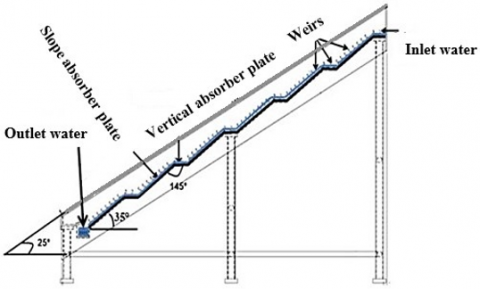Figure 1. The improved device structure diagram, (with slope surface and baffles) 

## 2.pngFigure 2. Three-dimensional representation of the prototype of novel cascade solar still 

3. Experimental Procedure

During the experiments, a tank of 25 liters of saline water alimented the cascade solar still. The salinity level was chosen at about 33.5–37.4 g/l. The used thermocouples aimed to measure the main parts temperatures of the still. Three thermocouples have been used to measure the glass temperature and six for the absorber plate. The experimental data were recorded for 11 hours, for each 1-hour time all temperatures at various points were measured, as well as the solar radiation intensity, and freshwater production.

Figure 3 presents a sectional view of a cascade solar still with baffles. The saline water temperature inside the solar still increases because the basin absorbs the radiations from the sun are refracted through the glass cover. consequently, the produced vapor condenses on the interior face of the glace cover. The water drops slip to the lower part of the glass cover. Thus, the distilled water is collected by a collector. The various temperatures and solar intensity have been measured using various instruments. Table 1 presents the precision and the error of the used tools.

## 3.png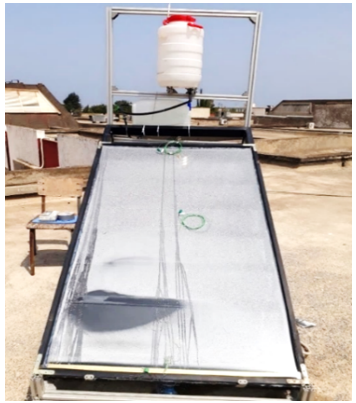Figure 3. Experimental set up of novel cascade solar still

Table 1. Accuracy and uncertainty of the measuring instruments 

 Instrument Accuracy Range % Error Thermometer +1℃ 0–100℃ 0.25% Thermocouple +0.1℃ 0–100℃ 0.5% PV type sun meter +1 W/m2 0–2500 W/m2 2.5% Measuring jar +10 ml 0–1000 ml 10%
4. Simulation

The evaporation and condensation processes were simulated using the volume of fluid model . The variation of the vapor interface is taken by the solution of a continuity equation for the volume fraction. The primary phase was indicated as water vapor and the secondary phase as liquid water with a volume fraction value of 1. In condensation and evaporation processes the mass transfer between the phases can be solved with a momentum equation with no-slip condition between the phases. The thermal model used was developed and coded by Bouzaid et al. .

4.1 Geometric and Meshing model

A structured mesh of hexahedral type was used containing a total of 2.8 million cells at a growth rate of 1.2, and a grid independence test has taken into consideration to examine the mesh size of the model to obtain more precise and reliable results . The three-dimensional model geometry of the cascade solar still with unstructured meshes is shown in figure 4.

## 4.png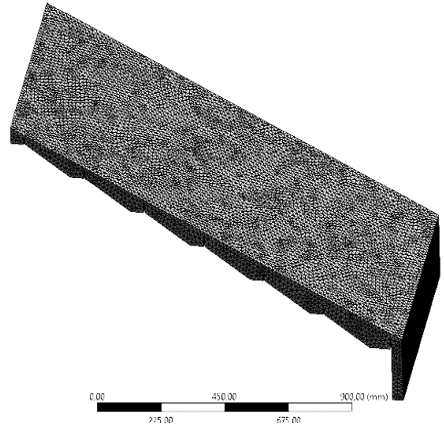Figure 4. 3D meshing for cascade solar still

4.2 Boundary conditions

Defining boundary conditions is an important step to attain an accurate solution. The conditions are specified from the experimental data, including the initial temperatures of the feed water, ambient air, and the amount of radiation received.

According to the computer restrictions and the high number of time steps and computer restrictions, the CFD simulation has required a high run time of about 10 hours. To simplify the calculation, a time period of 1 hour was taken and for every 1 hour of time interval, an average temperature was set as a boundary condition for the next simulation. The sidewalls boundary conditions were considered as adiabatic walls and the water outlet boundary condition was specified as the pressure-outlet condition. The five radiation models offered by ANSYS FLUENT for the heat transfer simulations included a radiation heat transfer, with or without a participating. Moreover, a solar load model includes the effects of solar radiation simulations. In this study, the radiation model selected is the Rosseland model with solar loading and solar ray tracing. The Rosseland model can be applied only for optically thick media and for optical thicknesses greater than 3. Moreover, The Rosseland model is cheaper, more efficient, and requires less memory.

The thermal properties of the variables used are given in Table 2.

Table 2. The thermal properties of the variables used

 Parameter Symbol Value Specific Heat of Glass Cover Cg (j/kg.K) 800 Specific Heat of Brackish Water Cw(j/kg.K) 4190 Specific Heat of Absorber Plate Cb (j/kg.K) 896 Specific Heat of Insulation Material Cins (j/kg.K) 670 Thermal Conductivity of Glass Cover λg (W/m K) 1.02 Thermal Conductivity of Brackish Water λw (W/m K) 0.67 Thermal Conductivity of Absorber Plate λb (W/m K) 73 Thermal Conductivity of Insulation Material λins (W/m K) 0.059 Density of Glass Cover $\rho$g (kg/m3) 2530 Density of Brackish Water $\rho$w (kg/m3) 1022 Density of Absorber Plate $\rho$b (kg/m3) 2700 Density of Insulation Material $\rho$ins (kg/m3) 200 Air Molecular Weight Ma (kg/kmol) 29 Water Molecular Weight Mw (kg/kmol) 18 Water Surface Absorptivity αw 0.9 Glass Cover Absorptivity αg 0.052

4.3 Assumptions

The assumptions used in the CFD simulation are:

- No vapor leakage from the destination unit.

- During the simulation the side walls were considered adiabatic.

- The air velocity was assumed negligible at the inlet.

- No temperature gradient across the glass cover and the absorber plate.

- The wind velocity was considered to be negligible because of the ambient wind velocity was low.

4.4 Mathematical formulations

In this research, to obtain a collection of discretized equations, The differential equations are integrated over an elementary control. The SIMPLE algorithm provides a coupling between the pressure and velocity expressions. For all equations, the convergence is satisfying when the values of residuals are reduced to 103. While the energy equation is considered value is determined at 106.

4.4.1 Governing equations

The governing equations of this problem are as follows:

• Continuity equation:

$\frac{\partial \rho}{\partial t}+\nabla \cdot(\rho V)=0 \quad \nabla \cdot(\rho V)=0$    (1)

• Momentum equations:

X-component: $\frac{\partial(\rho u)}{\partial t}+\nabla \cdot(\rho u \mathrm{~V})=-\frac{\partial p}{\partial x}+\frac{\partial \tau x x}{\partial x}+\frac{\partial \tau y x}{\partial y}+\frac{\partial \tau z x}{\partial z}+\rho \mathrm{fx}$    (2)

$\mathrm{y}$-component: $\frac{\partial(\rho v)}{\partial t}+\nabla(\rho v \mathrm{~V})=-\frac{\partial p}{\partial y}+\frac{\partial \tau x x}{\partial x}+\frac{\partial \tau y x}{\partial y}+\frac{\partial \tau z x}{\partial z}+\rho$ fy    (3)

$\mathrm{z}$-component: $\frac{\partial(\rho w)}{\partial t}+\nabla \cdot(\rho \mathrm{wV})=-\frac{\partial p}{\partial z}+\frac{\partial \tau x x}{\partial x}+\frac{\partial \tau y x}{\partial y}+\frac{\partial \tau z x}{\partial z}+\rho \mathrm{fz}$    (4)

• Energy equation:

$\frac{\partial}{\partial t}\left[\rho\left(e+\frac{\mathrm{v} 2}{2}\right)\right]+\nabla \cdot\left[\rho\left(e+\frac{\mathrm{v}^{2}}{2} \vec{V}\right)\right]=\rho \dot{q}+\frac{\partial}{\partial x}\left(k \frac{\partial T}{\partial x}\right)+$

$\frac{\partial}{\partial y}\left(k \frac{\partial T}{\partial y}\right)+\frac{\partial}{\partial z}\left(k \frac{\partial T}{\partial z}\right)-\frac{\partial(u p)}{\partial x}-\frac{\partial(v p)}{\partial y}-\frac{\partial(w p)}{\partial z}+$

$\frac{\partial(u \tau x x)}{\partial x}+\frac{\partial(u \tau y x)}{\partial y}+\frac{\partial(u \tau z x)}{\partial z}+\frac{\partial(v \tau x y)}{\partial x}+\frac{\partial(v \tau y y)}{\partial y}+$

$\frac{\partial(v \tau z y)}{\partial z}+\frac{\partial(w \tau x z)}{\partial x}+\frac{\partial(w \tau y z)}{\partial y}+\frac{\partial(w \tau z z)}{\partial z}+\rho \vec{f} \cdot \vec{V}$    (5)

• Volume conservation equation:

This is simply the constraint that the volume fractions sum to unity.

rG + rL = 1    (6)

4.5 Energy balance

This section presents the main equations of the thermal energy balance of cascade solar still have been used in the analytical model. The overall interpretation of expressions is explained by Bouzaid et al. .

• Thermal energy balance of the glass cover

Cpgmg$\frac{\mathrm{dTg}}{\mathrm{dt}}$= Qrwg + Qcwg+ Qewg − Qrgsky − Qcga + Pg    (7)

• Thermal energy balance ᴏf the brackish water:

Cpwmw$\frac{\mathrm{dTw}}{\mathrm{dt}}$= Qcbw− Qrwg− Qcwg− Qewg+ Pw    (8)

• Thermal energy balance ᴏf the absorber plate:

Cpb mb$\frac{\mathrm{dTb}}{\mathrm{dt}}$= − Qcbw– Qcd + Pb    (9)

• Thermal energy balance equation of the inner face ᴏf insulation material:

Cp,insMins $\frac{d T i, i n s}{d t}$ = − Qcd,ins+ Qcd    (10)

• Thermal energy balance equation for the external face ᴏf insulation material:

Cp,insMins $\frac{d T i, i n s}{d t}$ = − (Qr,ia+ Qc,ia) + Qcd,ins    (11)

5. Results and Discussion

CFD simulation of cascade solar still has been carried out and the obtained results presented in this section. the validation of the model was carried out by comparing with the experimental data and analytical model. The experimental results were conducted on July 14th, 15th, 16th 2018 in meteorological conditions of RABAT - Morocco. The analytical model was coded by using the energy balance equations.

Figure 5 shows the solar intensity behaviors from the morning until reaching its maximum at around 2 to 3 pm. Afterwards, the solar intensity drops by the end of the afternoon. The solar radiation measures on 14th, 15th, 16thof July 2018, achieving a maximum value of 887 w/m2, 923 w/m2, 773 w/m2 respectively.

Figure 6 shows the ambient temperature obtained from experimental results. The ambient temperature collected on the 14th, 15th, and16th reaches its maximum of 34℃, 37℃, 35℃ respectively.

## 5.png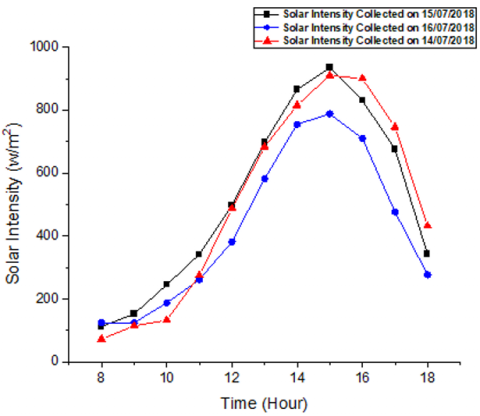Figure 5. Measured global radiation intensity

Figure 7 depicts the experimental results taken on July 14th, 15th, 16th of 2018. Due to higher absorption of solar radiation at the afternoon, the absorber plate temperatures reached a maximum of 60℃, 62℃, 57℃, respectively. Hence, the temperatures at the end of the afternoon decreases since the heat loss occurrence in the cascade solar still. The heat equilibrium achieves due to the continual contact between the absorber plate and the saline water, that signify their temperatures are contiguous. The maximum water temperature values achieved are 58℃, 60℃, 56℃, respectively. The absorber temperature presents the same behaviors of the water temperature. It increases during the morning till achieving its maximum. The curves of the analytical results track the same tendency of the experimental results. However, the CFD simulation presented an accurate result better than the analytical data in comparison with the experiments.

## 6.png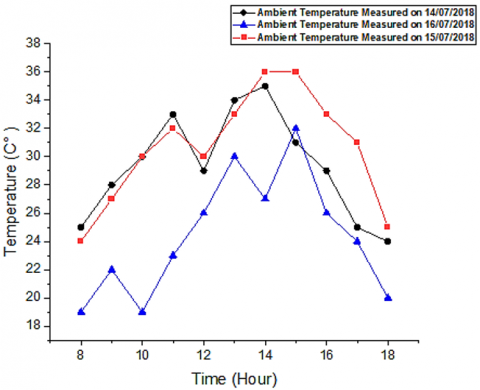Figure 6. Hourly variation of ambient temperature measured on July 14-15-16 2018

## 7a.png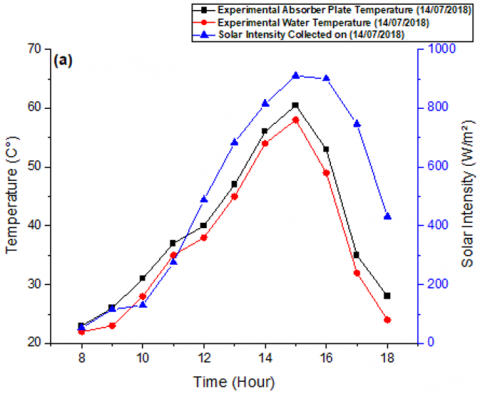## 7b.png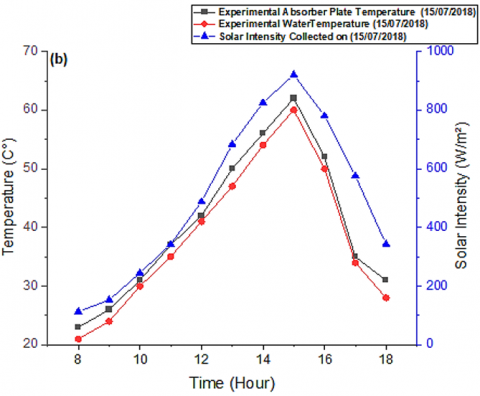## 7c.png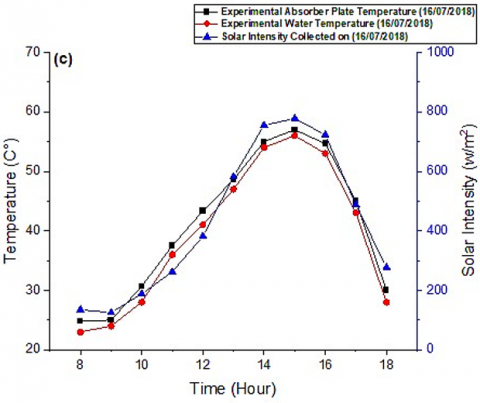Figure 7. Hourly variation of solar radiation, water temperature and the absorber plate temperature measured on (a) July 14th 2018, (b) 15th 2018, (c) 16th 2018

Figure 8 illustrates the hourly variation during experiments for the water temperature. the results indicated that the water temperature growths from 8 am until reaches its maximum at 3 pm then falls. Moreover, the water temperature has the same direction of solar radiation intensity. The CFD simulation and the experiments results are following a similar attitude. However, the analytical results not perfectly match to the experimental data, while the CFD simulation presents a total match form.

## 8a.png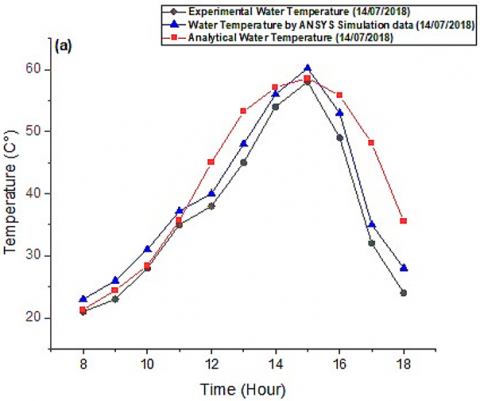## 8b.png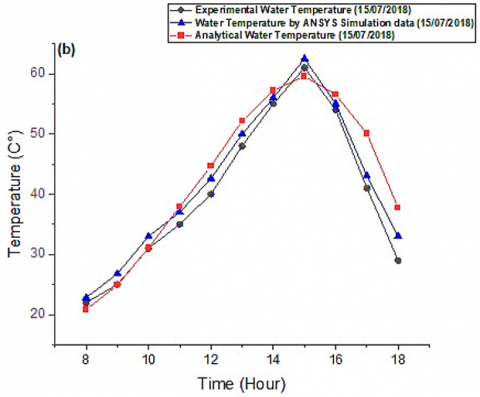## 8c.png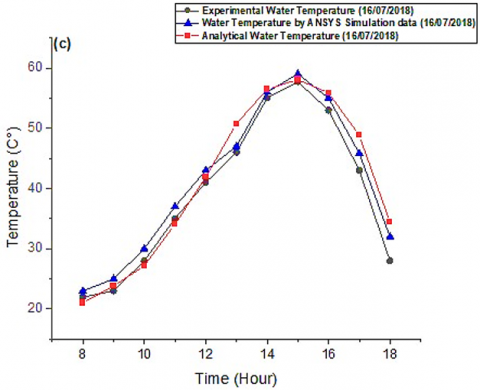Figure 8. Hourly variation of the CFD simulation, analytical and the experimental water temperature. (a) July 14th, (b) 15th, (c) 16th 2018

Figure 9 shown the productivity variation during the experiments as well as the CFD simulation and analytical results. the increases of solar intensity during the day leads to boost the heat and evaporation inside the still. consequently, the productivity is increased during the period where the solar intensity presents a higher value. The production of distilled water increases during morning until it attains the maximum at 3 p.m. subsequently, the productivity drops as well as the solar intensity. According to the first day of experiments, the productivity of fresh water reaches about 1.65 kg/ m2hr for 887 w/m2 of solar intensity (July 14th - Figure 9a), the second day it was about 1.69 kg/ m2hr for 923 w/m2 (July 15th - Figure 9b), and on the third day (July 16th – Figure 9c) it was approximately 1.37 kg/ m2hr for 773 w/m2. The results show that the CFD simulation, experimental data and the analytical solution are in good accordance which ensuring the mathematical model accuracy. However, the experience and the simulation results present a dissimilarity as a consequence of the assumptions in the models and the energy losses in the solar still.

Figure 10 shows the volume fraction of all three phases. It was showed that initially, the volume fraction of air was too high because of the absence of vapor. When the temperature increases, the volume fraction of vapor increases, and that of air decreases significantly.

## 9a.png## 9b.png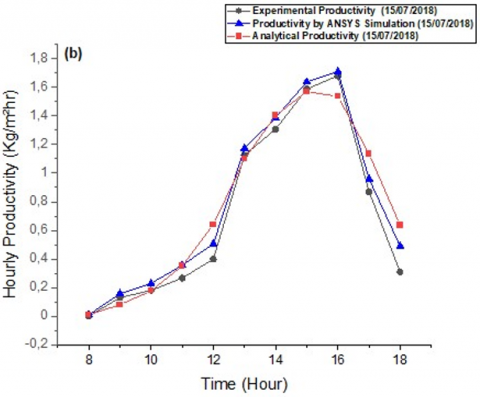## 9c.png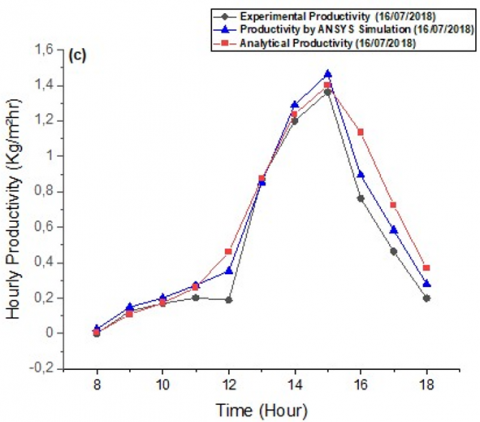Figure 9. The hourly productivity of the cascade solar still. (a) 14th, (b) 15th, (c) 16th 2018

## 10.png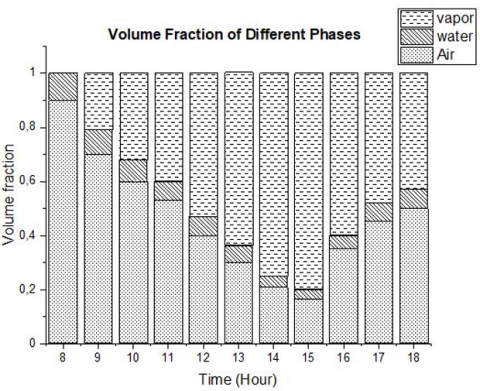Figure 10. Overall volume fraction of different phases

It was observed that the results obtained by Computational Fluid Dynamics simulation and available experimental data are good in agreement. According to the comparison between the experimental results and the CFD simulation, the relative error was 2%, while the relative error between the experimental and analytical results was 5.18%. The distillate output is proportionate with solar intensity where the second day (July 15th) of experiment presents a higher productivity in comparison with the other days. Due to high solar intensity, the condensation rate is raising inside the cascade solar still.

6. Conclusion

In this paper the performances of a new design of a solar still have been examined by three approaches, an analytical model a CFD simulation using ANSYS FLUENT®, and experiments. The first approach has been the use of a simplified mathematical model developed in previous work (Mouhsin et al. ). The results obtained by this simplified model showed quiet quality and capability performances compared to the use of complete CFD computations. All the results obtained by the two first approaches are compared to experimental data collected on a prototype developed within the working team at ENSAM Rabat.

Based on the result of the present work the main conclusions are as follows:

Experimental data permitted to validate the simulation results of water productivity, water temperature, and absorber temperature with the. It was observed that the CFD simulation as well as the analytical model results are in good agreement despite minor errors. The most probable reason for this difference is the simulated values are not concerned to the natural attenuation that typically happen in the experiments.

The results indicated that the productivity improves when the absorber plate temperature rises. Therefore, a high absorber plate temperature is the most significant factors affecting the productivity. The thermal performance of the cascade solar still was improved due to the new elements introduced to the new structure of the absorber plate.

During the 3 days of experiments, the productivity achieved an important values 6.74 kg/ m2.day on 14th July, 7.86 kg/ m2.day on 15th July, and 5.55 kg/ m2.day on 16 July.

NOMENCLATURE
 A Area, m2 Cp Specific heat, J Kg−1°K−1 h Heat transfer coefficient, W m-2K-1 IG Incident solar power, W m-2 Q Heat flux density, W m-2 m Mass, kg t Temps, hour δt Calculation step, hour T Temperature δT Incremental rise, °C ΔT Temperature difference, °C Greek symbols $\mathcal{E}$ Emissivity α Absorptivity τ Transmissivity λ Thermal conductivity, Wm-1K-1 Subscripts a Ambient b Absorber c Convection cd Conduction e Evaporation g Glass r Radiation sky Sky w Brackish water
References

 Reddy, K.V., Ghaffour, N. (2007). Overview of the cost of desalinated water and costing methodologies. Desalination, 205(1-3): 340-353. https://doi.org/10.1016/j.desal.2006.03.558

 Kabeel, A.E., Khalil, A., Omara, Z.M., Younes, M.M. (2012). Theoretical and experimental parametric study of modified stepped solar still. Desalination, 289: 12-20. https://doi.org/10.1016/j.desal.2011.12.023

 Alaudeen, A., Johnson, K., Ganasundar, P., Abuthahir, A.S., Srithar, K. (2014). Study on stepped type basin in a solar still. Journal of King Saud University-Engineering Sciences, 26(2): 176-183. https://doi.org/10.1016/j.jksues.2013.05.002

 Abdallah, S., Badran, O., Abu-Khader, M.M. (2008). Performance evaluation of a modified design of a single slope solar still. Desalination, 219(1-3): 222-230. https://doi.org/10.1016/j.desal.2007.05.015

 Muftah, A.F., Alghoul, M.A., Fudholi, A., Abdul-Majeed, M.M., Sopian, K. (2014). Factors affecting basin type solar still productivity: A detailed review. Renewable and Sustainable Energy Reviews, 32: 430-447. https://doi.org/10.1016/j.rser.2013.12.052

 Ayoub, G.M., Malaeb, L., Saikaly, P.E. (2013). Critical variables in the performance of a productivity-enhanced solar still. Solar Energy, 98: 472-484. https://doi.org/10.1016/j.solener.2013.09.030

 Rashidi, S., Bovand, M., Rahbar, N., Esfahani, J.A. (2018). Steps optimization and productivity enhancement in a nanofluid cascade solar still. Renewable Energy, 118: 536-545. https://doi.org/10.1016/j.renene.2017.11.048

 Benhadji Serradj, D.E., Anderson, T.N., Nates, R.J. (2021). The use of passive baffles to increase the yield of a single slope solar still. Solar Energy, 226: 297-308. https://doi.org/10.1016/j.solener.2021.08.054

 Ranjbaran, A., Norozi, M. (2019). Design and fabrication of a novel hybrid solar distillation system with the ability to brine recycling. International Journal of Heat and Technology, 37(3): 751-760. https://doi.org/10.18280/ijht.370311

 Norozi, M. (2017). Experimental investigation of improving received radiation by an hourly sun tracking on a weir-type cascade solar still. International Journal of Heat and Technology, 35(4): 737-746. https://doi.org/10.18280/ijht.350407

 Mouhsin, N., Bouzaid, M., Taha-Janan, M. (2021). Improving the cascade solar still performance using baffles fixed on the absorber plate: Experimental study. International Review of Mechanical Engineering (I.RE.M.E.), 15(8). https://doi.org/10.15866/ireme.v15i8.21020

 Moungar, H., Azzi, A., Youcef, S., Aabdelkrimr, H. (2018). Monthly fresh water yield analysis of three solar desalination units a comparative study in the south Algeria climatic condition. International Journal of Heat and Technology, 36(4): 1330-1335. https://doi.org/10.18280/ijht.360423

 Moungar, H., Azzi, A., Youcef, S., Aabdelkrimr, H. (2017). Immersed fins influence on the double slope solar still production in south Algeria climatic condition. International Journal of Heat and Technology, 35(4): 1065-1071. https://doi.org/10.18280/ijht.350444

 Sathyamurthy, R., Nagarajan, P.K., Edwin, M., Madhu, B., El-Agouz, S.A., Ahsan, A., Mageshbabu, D. (2016). Experimental investigations on conventional solar still with sand heat energy storage. International Journal of Heat and Technology, 34(4): 597-603. https://doi.org/10.18280/ijht.340407

  Bouzaid M, Oubrek M, Ansari O, Sabri A, Taha Janan M. (2016). Mathematical analysis of a new design for cascade solar still. FDMP, 12(1): 15-32. https://doi.org/10.3970/fdmp.2016.012.015

 Hirt, C.W., Nichols, B.D. (1981). Volume of fluid (VOF) method for the dynamics of free boundaries. Journal of Computational Physics, 39(1): 201-225. https://doi.org/10.1016/0021-9991(81)90145-5

 Bouzaid, M., Ansari, O., Taha-Janan, M., Oubrek, M. (2018). Experimental and theoretical analysis of a novel cascade solar desalination still. FDMP, 14(3): 177-200. https://doi.org/10.3970/fdmp.2018.00330

 Mouhsin, N., Bouzaid, M., Taha Janan, M., Oubrek, M. (2020). Modeling and experimental study of cascade solar still. SN Applied Sciences, 2: 708. https://doi.org/10.1007/s42452-020-2521-x

 Bouzaid, M., Ansari, O., Taha-Janan, M., Mouhsin, N., Oubrek, M. (2019). A numerical analysis of thermal performances for a novel solar desalination still design. Energy Procedia, 157: 1071-1082. https://doi.org/10.1016/j.egypro.2018.11.274